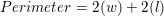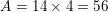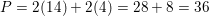# SSAT Middle Level Math : How to find a rectangle on a coordinate plane

## Example Questions

### Example Question #1 : How To Find A Rectangle On A Coordinate Plane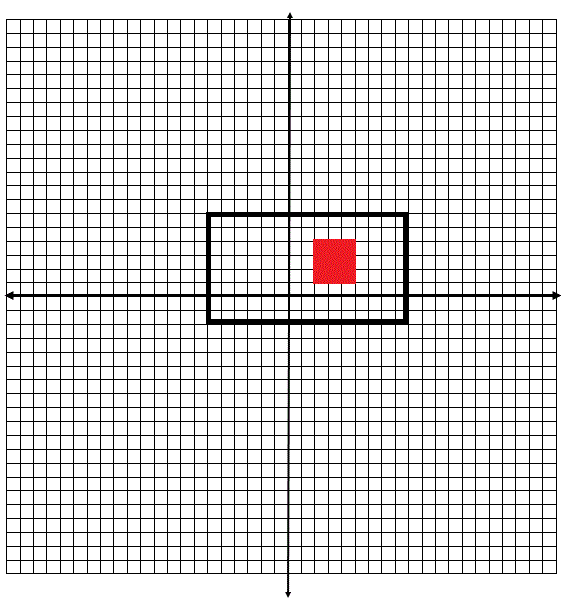In the above figure Dr. Robinson's shed is represented by the red square, and the shed has a perimeter of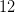yards. Find how much area Dr. Robinson has in his backyard--excluding the area that the shed occupies.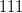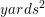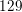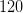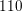Explanation:

To find how much area Dr. Robinson has around his shed in his backyard, first apply the formula: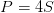, where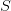represents one side of the red shed. Given that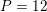must equal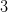because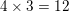.

Now you have enough information to find the area of the red shed.
Apply the formula: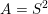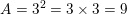Since the rectangular fence has a width of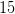yards and a length of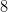yards, apply the formula: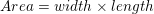in order to find the area of the entire backyard.
Thus,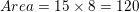Then simply find the difference between the area of the entire yard and the area of the shed.

Thus the final solution is: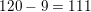### Example Question #2 : How To Find A Rectangle On A Coordinate Plane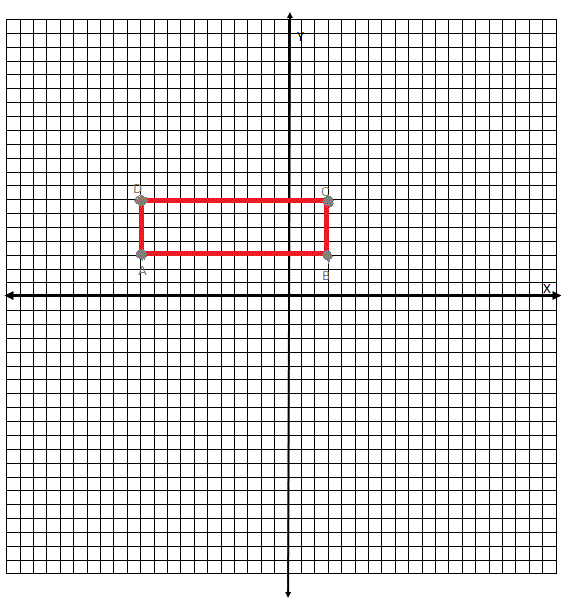Identify the correct set of coordinates for rectangle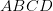.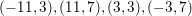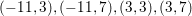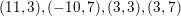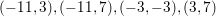Explanation:

To identify the correct set of coordinate points for rectangle, note that the correct set must have two sets of matchingcoordinates and two sets of matching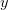coordinates. The only answer choice that meets these specifications is:### Example Question #3 : How To Find A Rectangle On A Coordinate Plane

Dr. Robinson recently put a rectangular fence around his backyard. The fence has a width ofyards and a length ofyards. If Dr. Robinson paid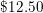for every yard of fence, how much did the fence cost?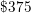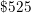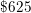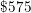Explanation:

To find the cost of the fence, apply the formula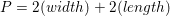in order to first find the length of the perimeter of the fence.

Then multiply the perimeter by.

Thus, the solution is: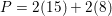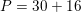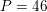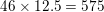### Example Question #4 : How To Find A Rectangle On A Coordinate PlaneThe rectangle shown above has a width of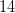and a length of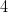. Find the area and perimeter of the rectangle.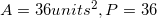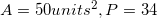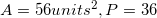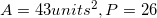To solve this problem apply the formulas:&